# Appendix A Thevenin`s Theorem - Department of Physics | Oregon

## Transcription

Appendix A Thevenin`s Theorem - Department of Physics | Oregon
```Appendix A Theve nin's Theorem ,,- - -)
!,
,
I
- ----- - -- " ' ,
13
:,
~_.
_____ . '
Any network can be described by loop currents passing from volt­
age sources to the terminals AB, hence through a load resistor RL.
An example is shown in figure A .I. In order to establish Thevenin's
theorem, it is necessary to show that V AB = VEQ - REQ lAB, where
VEQ and REQ do not depend on the load resistance RL·
Around each current loop, there is a linear equation:
= VI VA.B = V2 -
VAB
RllIl - RI2h R21Il -
... -
Rlnln
R2212 - . . , - R 2n l n
Fig. A I. Loop currents
through termInals AS.
Because the voltage drops appearing on the right-hand side in the
form ~j Ij stop short of the terminals AB , the load resistance RL
does not appear explicitly anywhere in the equations. They can be
solved for currents (by simple elimination and substitution), with
the results
= Y1I VI + Y12V2 + ... + Yin Vn 12 = Y21 VI + Y22 V 2 + .. . + Y2n Vn -
It
/1 VAB
l
/2 VAB
where again none of the coefficients depend on RL (since ~j did
not). Then hB = Li Ii can be written Bl VI + B2 V2 + ... +
Bn Vn - CVAB . This is of the required form if C = l/ REQ and
VEQ = (BI VI + B2 V2 + ... + En Vn)/C.
In Chapter 6, it is shown that there is a linear relation between
V and I for capacitors and inductors when the notation of com­
plex numbers is used. The linear relation between V and I is the
foundation of Thevenin's theorem. Hence the proof carries over to
include capacitors and inductors in AC circuits. Fourier's theorem
expresses any waveform in terms of AC components, and Thevenin 's
theorem is therefore generally valid for any network where the re­
lation between V and I is linear. This extends it to small signals
in non-linear circuits, following the methods of section l.ll.
354
1
e
s
E
v'
w
®
A \~
w~ tL
to
0-
Q,
+w
CA
V{
~,
J""\A.-.3-\~ CI~
~ v-\t~~.,UZ &.. 'i·-e.s-~ ~-ta...\J\O-s
1
~v LA"':AV'-a1<;.. ~I S e Ie. ct", eaJ
9 ~ 1.<..-, \kJ,i1
'l ~ eat
c.. tAo'V \H2- ~ ~ u. \J U- "-I \.....,
" <"l ~'e.r w ~~ l
d
S\ (". to..vv~
);.
0
:
A.
iAtVvVv-o
\f ---"-­
L----!
= I.-~ (r-~.~;... /
o .... (~~ ..... p
:::­
®
v
i
L
' j
:::
\J~ ~t:
IL
I
, ) 1./
Ta
"'-.)'l,i')
'l'
..!..L
('''''''l.
--
.~
•
f'
-1...
'14)
G
.'-"
~
'­
t,. 'I"", .:..,
"7
/
-rf
tA.V1' JP...;­
)J,..':f.~,
~\
')-'~
,
I~
-I.­
-­
'!
"­
- ­"
'0
s=R
\j
t
t
wcu.
p\JCi.-J-~c.
~c
-to
~
v t'. ~'6
1-0
\f'\. G
~
-: '1~\9.., ~Xt1-IA.L\')"'--! 1 ~ t
sfQj' \,;~~.
'2...:::;> V\-
V~~ L"~'«cl
~t w.~
.
c·. e. C. _
~~
~~
+\
<;
t
CL-
cuJ
~CC~
~\)'~~ld{t\
U E 55"1 t>""'oc~: (a-f
Fcrcvv'"\ oJ.
I
'-V\'\' _ \/ Rl- (0 n'1\ V\~)t ~
L -
c..' IJ'cU:~
R-t
3t: V~, ·R
,-.
.
,
Qv...~1GcV'w = -10~ w~ol
\fOv\~S
I~
=I~
If
-
Rtf: l
t
~ ~~ "J,\,\tS
~,~ ' I t~~ """~~ - ~Q.V\~ S,c\~5
I
~ ~\A)~s.
1i
I
e9~
i
V\II.~vr
R l J.. S ~
W)
'W~
, . -\~ vCL\1JJU
0\;
1 '1.
13...
+ ~ 'Rl =-..1 1. 1< \. .
y
V
I z \'.. I,
'f"'."
.
f.i:
,
+ R~ t\"e.IrI:
•
(t~~().A,~i., \1 'l/t, '"
% 1~~
I
N0i~ f~J ~e)1~ s(~t~
£
\A,Vl
('k~ a~ S ,
"3'-\ -fex)::
\ (,,)
~ ~()()
0~
Q
I
;
01
uho
#
~/o.. '" o/'G _)
-l~e..- e.1u.J{~( C0~
L, \')LtW
RL.
x -+ b J
-t-t
<MA
~ ()() -= C)( +
N\~!~ he..:
*tnttlr./ J ~ {
v..~ 6 -=- ~
,.
Ov = C
~ 6 8< ~ CN~
Q
&C
"
; \l\1t\J~~~cw fGi\Ut~
\$\o~
>
A
r-) \ ,
~Ld\~,
t).L-- ft
--."...".
t
; -
c? :rv~ e.,... (I.uI.i\V
l
-
'IV'
~ 10cl'L.i t)'·.
-f\ '.,:"­
"
Cl.JJ-,
~~v~~ \)-C~C<;tOL~:
\ ........;_
A
11/)\ ,
-L
-
C. ,)
r------~Atv~~
,
. . _.
~ t -'
\
<.
Iy
Vvvl> \)-C,
~l-:
__
I
-­ _1 _--~ t­
c-\o
c.
~
t J ')
CL
(l v..\I.t.
f
O u..,..r
\.1'(.V"7 (}·WV....
1'\.
t
t'r~""'~! LL-t vL<;
'-0<;; ~~
Q \~ t'-fct.1-y
13 J- 1
hr
,' r
0...,
f.s~~I<J
A
s Q..Wv
<
--..L.
V ­
1::>
1?
0..1.
J,
:~
l
r
J~f
,
.\.....
*
!,..;
~J.;~
L
~
'il~
e,"'t
-t ~, tv..'\. -
.....
~
,
0-1.
~ .)\rv
1
c:\ I
..ll ~ ,<v c..
I'.:'
~
t- \.- '
c-
..;'1
Ity
.lL
e
'1':
-
I..l '
-
\lC,UA~
II..... -t~
~
<I
~
tA.
\' ' t
-
,,-
•
J
, j :.2 _"".):7
'..J
~
"C'~
> ----.
'"
...
i K
L
,
•
----
-
ov\. J -
=
I
L
-, \I"V~0:
",--t; V- v..., (.., c\. :
:
­
V
~_ _ _ _ _ _ _ __ _
L
( ~(,..j
N r/' 'U
C0
t
R) -+ ~ L
tVv~ CJ~
l~ :
'f" 1:
-=
«\J: + 1(..) & 1. ?=\; ~-tl.
~~
10~l.:> w\" J - ~"'e.-JJ~o,,-\o;' f1~~Jt.j "
f"
c Cv~ e\$o J
~ Q....\N-<.-
t<"l
~·~~\'·~.5f I~
kJL
,\0T t~
\v {.,
V
T ~e..\>-01
1 dtlA...,
l.L
I \..v
cv
S; '\ ~
('/V<- :
I./'---t!J
A
~
v
I
~~
t r.Ji-L~
~ct
'2.
rut
R::=
0 ;
1'­
y
I
.:::­
5
\", -\­
l V\ \
~
__~____~--.~A
1
(
I
1\ R" \\ R L
]
)
L.
=
\
- . \
-::--:­
.. L'
I
)
l''''~ cVv~
r l 't '" luaA.
IKS~qc<
T
t <; :
N Ow we..... ~-e.,~~
=
a
0
Gt. '\
~i0
cJ..\{~Vc~
to
1- .~it Ou.S
-. ­
-
I
J... L
1
Di ~ 'I
. T"-·K
i
I
R,-
i/
(~/ + f: If)
A
~ ~CN
TVlc.,,\r
fY,(J
"';. r-J
.Q,~ \(j~ vcJ(·j
v', \tc.. _, we..,. WQ j '.
~J
R/
R' t R I;
!he~io~~
I
JeD
•
-
-
\
~1
. -v -e..v-':
--) "V
~
~
"\J
Q.\J-
~
A
\tJ ~\'
®
\ Lvru:;I
lJ-eJ\ 7 ~GC(\.;u,Sv
I-Jc;L-'
;J \
'-I0'~' h
v.,
S1-" v ~ 1-..Y-­
C
G
c-~ ~~, -,1
tv ~
~~ GJ.~s
\ 'i 1). V_ \3 "'~ I> ( 0\..
V\).
~
"[ s s \"J,..
e.e\
C'IA (.L.
v..-(
(.j.
~I ~ t~ \>~
1/
I
q\\
h -\-l.-v s to ~R.A" ~1" w} Fl"x' "t -\ \...u
'11
f
v..~' ""t -\-"'-., \( , \0,
\j'
of\ ~
La...",:;·
Anot her worked example
Figure 1.19(a) shows a rather complicated circuit. As an illustra­
tlOn of all the methods which have been developed in this chapter
we shall fi~d all the currents and the voltages VA and VB at node~
by three dIfferent methods.
fig1
is
fhe I kO
O.6mA 15
I kO
I kO
B
Rs
R}R4
R)R Z
R} + P4 p) + R2
cb
t
2kO
=3kO
I}
=1 kO
+
VB
'2v
O.SmA
O.7S mA
2kO
12kO
6V
IOV
(a)
12kO
(b) 14 =0 . I SmA
1}= -04SmA
he
~r-
Fi g. i . 19 Worked examp I e
is
,
t
-
10V
(c)
12 =+0.2Sm A
I) =- O.2SmA
14
Voltage, Current and Resistance
Using superposition, the currents may be obtained from those
due to the individual batteries. In (b), the 10 V battery is shorted
out and R3 and R4 appear in parallel. The top three resistors
of (b) provide 2 H2 in parallel with 4 kO between A and earth,
i.e. ~ H2, so the contribution to II = 1.8 rnA. It is easy to see
how this divides at A; then h splits between 0.15 mA through R4
and a contribution of -0.45 mA to h In (c) , the 6 V battery
is shorted, so Rl and R2 appear in parallel. The arithmetic of
the resulting currents is shown in the figure. The signs of the
contributions to II and 12 are easy to follow from (a) and the
sense in which the 10 V battery drives currents. Adding currents
from (b) and (c), II = 1.55 mA, 12 = 1.45 mA, h = -0.7 mA ,
14 = -0.6 mA and Is = 0.1 rnA. From these currents, it is simple
to find VA = 6 - 3.1 = 2.9 V and VB = 10 + I4R4 = 2.8 V .
Suppose instead the problem is to be solved using mesh cur­
rents . The ones to choose would be 11 , 14 and Is, as shown in (a).
Applying Kirchhoff's current law, h = 12 + Is and 14 = h + Is ·
The values given in the previous paragraph satisfy these relations.
Then applying Kirchhoff's voltage law to each loop in turn:
6 =2h +2(11 - h)
= 4h -
21s
10 = - 1214 + 4(15 - 14) = 415 - 1614
o = lIs + 4(15 - 14) + 2(15 - h) = 715 - 414 - 211
with currents in mAo Solving these three simultaneous equations
is tedious. It is however straightforward to substitute the values
derived above and demonstrate that the equations are correctly
satisfied . Using superposition is really a graphical way of eliminat­
ing variables from the simultaneous equations.
The third alternative is to use node voltages V<\ and VB. Then
current conservation at these nodes gives
L
Ii
b
c
t,
e.
n
u
v'
it
ir
a:
Cl
OJ
v,
E
fc
ti
T
(f.
W.
The solution of these two simultaneous equations is easy; a check is
that the equations are satisfied by the values of VA and VB obtained
above.
ar
T)
1.1 0·
Non-linear Elements in a C ircuit
Superposition is a valuable shortcut , but (as demonstrated below)
it only works exactly for circuits containing linear components like
resistors, where Vex: I. The next chapter develops other powerful
shortcuts which again depend on linearity. However, many elec­
tronic devices such as diodes and transistors do not obey Ohm's
101
re
ca
sh
Norton 's Tbeorem
h
11
t
e
:-
II
f
27
(ii) Wi th the batteries replaced by short circuits, REq is the
resist ance across AB , namely 5 kD in parallel with 10 kD. So
REQ = 10/3 kD.
(iii) As a check, consider the situation with AB open cir cuit. In
this case, t here is a net voltage of 10 V in ( a), driving current I
in t he direction of the arrow through the 5 and 10 kD resistors;
I = 10/15 rnA and VAB
10 + 51
10 + 10/3 = 40/3 V. This
agrees wit h hqREq from (i) and (ii).
A circuit can often be simplified quickly and neatly by swopping
backwards and forwards between Thevenin and Norton equivalent
forms. T his is illustrated in figures 2.1l(b)-(d). It is a trick worth
pr ac tising, since it often saves a great deal of algebra. The batteries
and resistors of (a) are replaced by equivalent Norton circuits in
(b); t hese are combined in parallel in (c) and then (d) converts
back to the Thevenin equivalent form . An important warning is
that you must not include the load resistor between terminals A
and B in these manipulations : Thevenin's and Norton's theorems
app ly to the circuits feeding terminals AB.
=
=
Fig.2. 12. A worked exa mp l e
Rs
(al
Another worked example
F igure 2. 12 reproduces a fairly complicated example from Chap­
ter 1, fi gure 1. 19( a) . If all currents and voltages in the circuit are
required , it is best to use one of the methods from Chapter 1. Sup­
pose , however, only current 12 is to be found. It can be obtained
straightforwardly by application of Thevenin's and Norton's theo­
rems . The steps are shown in figure 2.1 3. In (b), V2 and Rt are
replaced by their Norton equivalent. Then R3 and Rt are combined
in par allel and (c) returns to the Thevenin equivalent form .
W ith AB open circuit ,
R]
R,
R4
Vj
V2
III
Rs
A B
R]
(bl
RI
W ith the batteries shorted out, R Eq is given by the parallel com­
bin ation of RI wi th
R5
+ R3R4/(R3 + R 4)
(e )
i.e . 2 kn in parallel with 4 kn, so REq = t4/3) kD.
As a check, the current through AB when shorted is 1Eq :
I
_ VI
Eq - Rl
V2 R 3
+ R3 + ~
(R
5
R3~)-1
+ R3 + Rt
= 3 + 2.5/4 = 29/8 mA o
Fig. 2 . 13. Worked exa mple
28
Tbevenin and Norton
re
This agrees with VEQ/REQ as it should . T he arithmetic and alge­
bra are sufficiently tortuous that this is a valuable crosscheck.
Finally, the current h of figure 2.12 is
V(
di
W.
th
go
in agreement with the value obtained in t he previous chapter.
Further examples are given in the exercises at the end of the
chapter. If you can do question 6, you have m astered the vital
points of Chapters 1 and 2 up to here .
2.
St
R:
to
2.5 General Remarks on Thevenin's and Norton's
Theore ms
Fi g 2. 14 For const ant Vou t ,
R EO << R L'
Fi g. 2 . 15. For constant
Rr:o » R L
lout,
B
(a)
(b)
Fig.. 2. 16. Wrong ch oi ces f or
equiv alent circuit s.
,­
(1) Suppose a constant voltage is required across a load R L ,
with as little variation as possible when RL is changed. From
figure 2.14, REQ needs to be small compared wit h RL, so that
mOlt of VEQ appears acrOls RL. Thus a constant voltage source
should h ave a low output resistance or output impedance, as it
is often called.
(2) Conversely, suppose a constant output current is required,
independent of load ; this is the case , for example, in supplying a
m agnet or a motor . From figure 2.15, this demands REQ ~ RL,
or high output resist ance.
(3) When a circuit is meas ured with an Olcilloscope or voltmeter ,
it is desirable to disturb the circuit as little as possible , i.e. draw
very little current. This requires the detector to have a high in put
resistance or input impedance. Oscilloscopes and multimeters
typically have input resistances of 10 6 - 10 7 n. On the other hand ,
if an ammeter is inserted into a circuit in order to measure current ,
we want to disturb the current as little as possible. Therefore an
ammeter should have a low resistance.
(4) Although T hevenin's and Norton's circuits are equivalent to
any network in the sense of giving t he same output volt age and cur­
rent, they are not equivalent as regards power consumption within
the equivalent circuit . You may easily verify that the power dissi­
pated in the Norton equivalent circuit of figure 2.10(b) is different
from that dissipated in the Thevenin equivalent circuit (a) . T his
is because power is non-linear in V or J.
(5) Common student howlers are to draw equivalent circuits in
the forms shown in figure 2.16 . It is wort h a. moment's thought to
see as to why these must be wrong. In the former case, VAB = VEQ
independent of load, which gives an absur d result if the terminals
are shorted. In the second circuit, J AB = IEQ independent of load,
and this is absurd if the terminals are open.
(6) If you encounter a circuit like tha t in figure 2.1 6(a) where
a resistor is applied directly across a bat tery, you can ignore the
R:
an
Tl
ml
of
no
so
sa
pc
ca
th
2.
In
h
m;
to
1
-~t:.-===-=-_ ---
w~~QJ dCJ'<\~
~, \r" ~ Q l,JAt c '
e.,,\ G
0... "-'
I
1::>v-~J~G - (\-"'- 01~\(V\J~ @ c- + . '" e- e,d IA.-C ..., ~VIOl" ~,~ ""I
V\.e,
~ ~: c.S '~
-rv
1
t
+~ ~"J,\/U \~ _~cJct-lAcel, +~ ;s IA.L­
clL"d f/o""y ~Lvoc
{k Vv.<Ji:w ; leD.,.-r K
-..5
k k, V l-t~f j v ~~ VA C = J \) ~
I
0-
d
0
\ 1 c - K2.1o
O.i~
Ie
c
f<~l~
5 (,I v ; ~
/+ £
1
1
D
'R 2. &. R4
0 ~&', tl ('
c... ~
k~
I
R-~
'=
~ ~~ I
e 9~ (,l v~ IN.
~ l;J
j D~
~
~
IA.-<;G
C;J
"
-1D
/1."" ,
Ow<.,
r
w <-
,J : ~
1:.\1\0 \.J'-'v
=
~l-t
R"l
,+w. 1&ro,....
Vv'
R)
\J.N
1:: k
=()
®
u ,. .
.L~
2.
-=:.
V- R + td~
(_+~
.
Jt
\AJe.,
w.,t,tQJJ I
o
j t'
-::4
~ ~ ~~~\$;4l\Jt.l
~.C\ .
y., V- ~J:.1i.J.r"
",-V-~w (t~ ~
c.o...u., ~wt.J ~~ s~\
t. ~ ```

### Alexander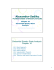### www.chlorofil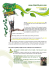### Thevenin`s and Norton`s Equivalent Circuit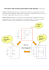### Chapter 20: AC Network Theorems### Thevenin equivalent circuits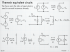### VS4874 SPU Anesthesia Breathing Circuit Brochure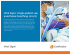### Depressed Center Wheels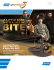### Experiment 17: Kirchhoff`s Laws for Circuits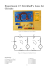### The Beat Goes On - Room 217 Foundation### Router High-Flex Green Efficiency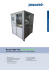### PDF - Inside Africa### Sitios de buceo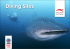### July 2016 - Masters Electrical Services, LTD### Parallel Operation of Turbo-Compressors in a Common System### Exercises Unit 5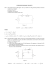### The one and only!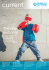### Introduction to the Design and Development of Mixed Signal### Newsletter - Town of Norton MA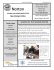### Isochronous synchronization in mutually coupled chaotic circuits### Device circuit breakers - the basics